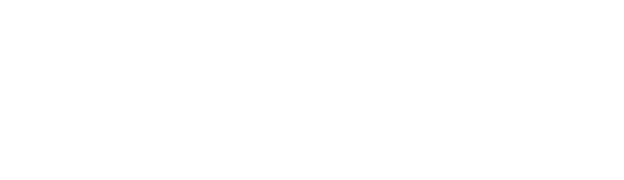# HDOJ：数圈圈

Problem Description

7111=0 5555=0
8809=6 8193=3
2171=0 8096=5
……
2889=？

（注意：4是没有圈圈的！）
Input

Output

Sample Input
7111
8809
2171
2889
Sample Output
0
6
0
5

```#include
#include
int main()
{
int num,t,tl,aws;
while (~scanf("%d", &num))
{

aws = 0;
if (num